Many readers ask the origin of the 63%.

The answer is found in examining the equation for the exponential charging process.  Assume that initially the membrane potential (Vm) is uncharged and then in response to some step stimulus, the membrane potential charges over time to a final value of 100% charged from its initial value of 0% charged.

This process can be described by the equation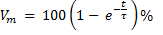Where t is time and τ is the time constant.

To illustrate the behavior of the equation, take some extreme cases. Assume time = 0. Then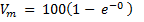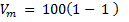Vm   =    0%

Now assume t = ∞. Then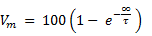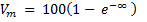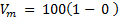Vm   =    100%

Now take the interesting case where the time equals the value of τ (i.e., t = τ)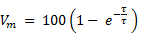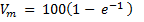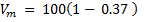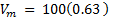Vm   =    63%

Mathematically, τ can be considered a constant that stretches or shrinks the exponential response in the temporal domain. As an exercise, try solving the equation with different values of τ.